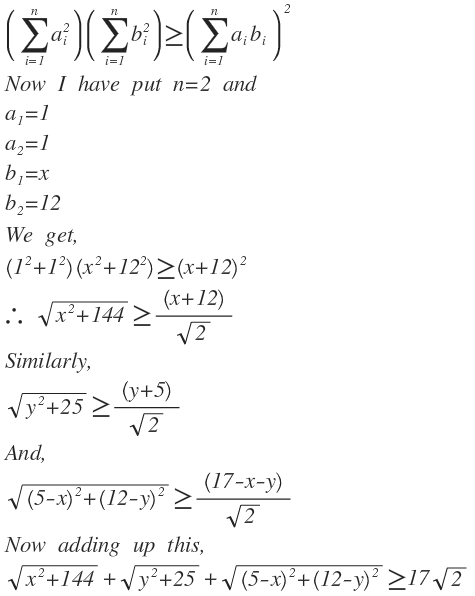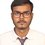# Cauchy-schwarz inequality.Raghav Vaidyanathan you should see this.Note by Trishit Chandra
5 years, 10 months ago

This discussion board is a place to discuss our Daily Challenges and the math and science related to those challenges. Explanations are more than just a solution — they should explain the steps and thinking strategies that you used to obtain the solution. Comments should further the discussion of math and science.

When posting on Brilliant:

• Use the emojis to react to an explanation, whether you're congratulating a job well done , or just really confused .
• Ask specific questions about the challenge or the steps in somebody's explanation. Well-posed questions can add a lot to the discussion, but posting "I don't understand!" doesn't help anyone.
• Try to contribute something new to the discussion, whether it is an extension, generalization or other idea related to the challenge.

MarkdownAppears as
*italics* or _italics_ italics
**bold** or __bold__ bold
- bulleted- list
• bulleted
• list
1. numbered2. list
1. numbered
2. list
Note: you must add a full line of space before and after lists for them to show up correctly
paragraph 1paragraph 2

paragraph 1

paragraph 2

[example link](https://brilliant.org)example link
> This is a quote
This is a quote
    # I indented these lines
# 4 spaces, and now they show
# up as a code block.

print "hello world"
# I indented these lines
# 4 spaces, and now they show
# up as a code block.

print "hello world"
MathAppears as
Remember to wrap math in $$ ... $$ or $ ... $ to ensure proper formatting.
2 \times 3 $2 \times 3$
2^{34} $2^{34}$
a_{i-1} $a_{i-1}$
\frac{2}{3} $\frac{2}{3}$
\sqrt{2} $\sqrt{2}$
\sum_{i=1}^3 $\sum_{i=1}^3$
\sin \theta $\sin \theta$
\boxed{123} $\boxed{123}$

Sort by:

I do not think that you can add the inequalities. The thing is that the three inequalities that you have added do not take minimum value for the same x and y. If all the three took minimum value for same x and y, then we could say that LHS is greater than or equal to 17root2. But now we can only say that it is strictly greater than 17root2.

Thank you for taking the time to put this up.

- 5 years, 10 months ago

Indeed. What he has shown is that $17 \sqrt{2}$ is a lower bound. However, he has not shown that this is the maximum possible lower bound, which would then be the minimum of the function.

For example, it is obvious that $\sqrt{ x^2 + 144 } \geq 0$ and $\sqrt{ y^2 + 25 } \geq 0$, which tells us that $\sqrt{ x^2 + 144 } + \sqrt{ y^2 + 25 } \geq 0$. But clearly, 0 is merely a lower bound of the expression, and is not equal to the minimum value of this function.

Staff - 5 years, 10 months ago

Ok I've understood this. And trying the problem to solve geometrically.

- 5 years, 10 months ago